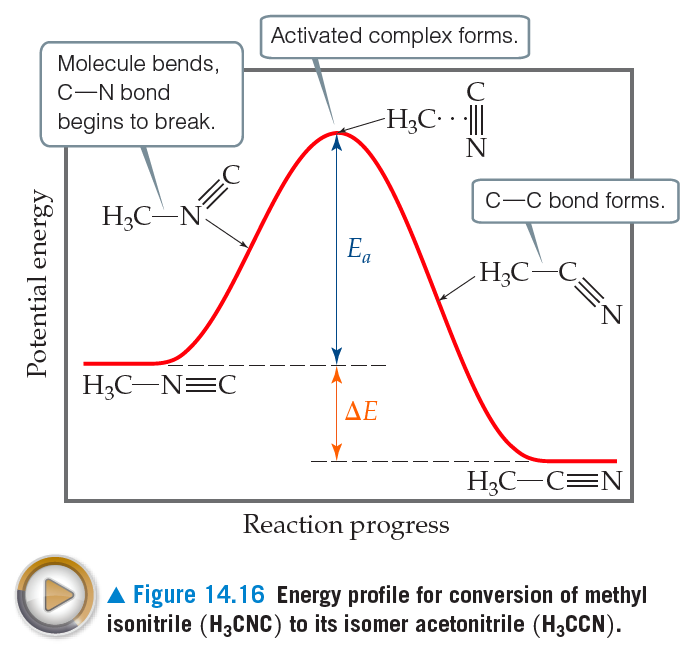# Problem: Suppose you could measure the rate constants for both the forward and reverse reactions of the process in Figure 14.16. In which direction would the rate constant be larger?

###### FREE Expert Solution

We are being asked to determine which direction for the reaction would the rate constant be larger

Recall that the rate constant or reaction rate is the proportionality constant that relates the rate of the reaction to the concentration of reactants.

82% (81 ratings)###### Problem Details

Suppose you could measure the rate constants for both the forward and reverse reactions of the process in Figure 14.16. In which direction would the rate constant be larger?Frequently Asked Questions

What scientific concept do you need to know in order to solve this problem?

Our tutors have indicated that to solve this problem you will need to apply the Energy Diagram concept. If you need more Energy Diagram practice, you can also practice Energy Diagram practice problems.

What professor is this problem relevant for?

Based on our data, we think this problem is relevant for Professor Albright's class at Thomas Jefferson University.